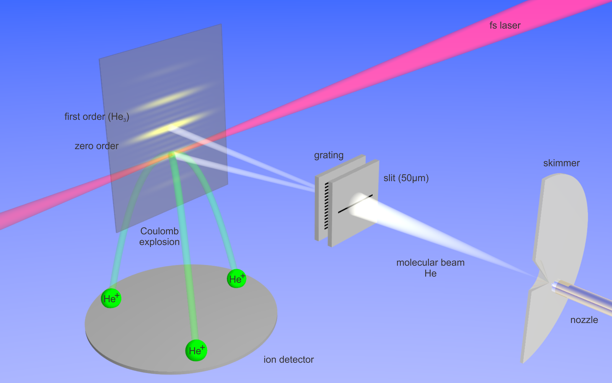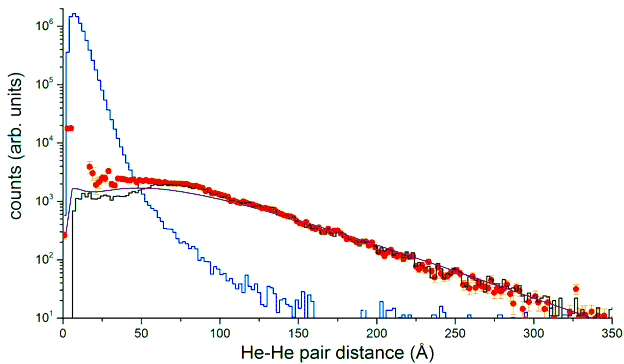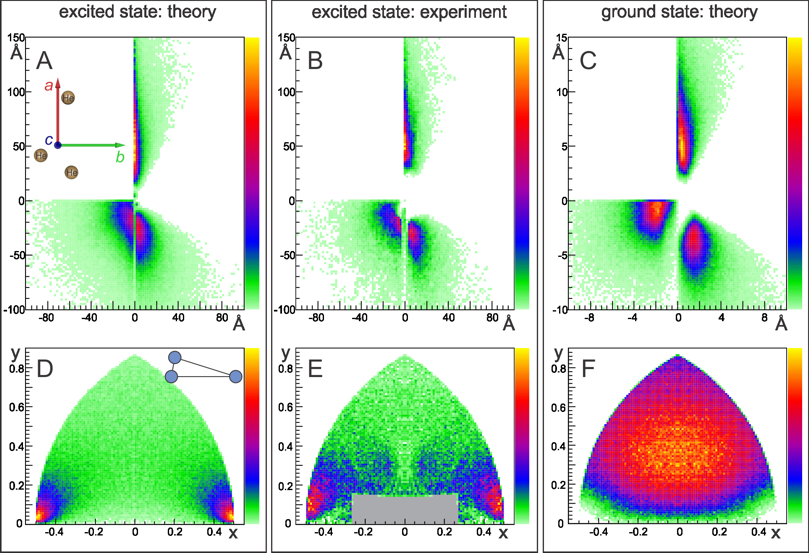﻿

## Observation of the Efimov state of the helium trimer

In 1970 Vitaly Efimov predicted remarkable counterintuitive behavior of a three-body system made up of identical bosons with a short-range two-body interaction. Namely, in the limit that each of the three pairs is just short of supporting a two-body bound state, the three-body system would support infinitely many three-body states with enormous spatial extent. This prediction gave rise to a new research field now called “Efimov Physics”. Very soon after Efimov’s prediction, it was suggested that 4He3 is the prime candidate for such a state. However, despite extensive experimental efforts, the Efimov state of the helium trimer has remained elusive so far.

In our laboratory by combining the wave matter diffraction with the laser induced Coulomb explosion imaging we have succeeded in detection of the excited Efimov state of the helium trimer, which was prepared under supersonic expansion of gaseous helium through a tiny nozzle (figure 1). The momenta, the helium ions acquire during Coulomb explosion, were measured by COLTRIMS. From these momenta the structural information about the excited Efimov state of the trimer was deduced using Newtonian mechanics to invert Coulomb explosion.Figure 1: Preparation and detection of He trimers.

Inter-particle distances of the Efimov helium trimer spread up to 300 Angstroms (figure 2). In average the excited state is about 8 times larger than the ground state. The geometry of the Efimov state is remarkably different from that of the ground state. Whereas the ground state corresponds to an almost randomly distributed cloud of particles, the excited Efimov state of 4He3 is dominated by configurations in which two atoms are close to each other and the third one farther away, in the classically forbidden region of the two-body interaction potential (figure 3).Figure 2: Pair distance distributions Ppair(R) of the excited (red, black) and ground (blue) states of He3. The theoretical pair distance distribution of the excited helium trimer is shown in purple.Figure 3: Structures of the helium trimer. A, B respectively show the theoretical and experimental excited state structure; C shows the theoretical ground state. Note the different scale for the ground state structure (C). For the plots the center-of-mass of the trimer was shifted to the origin. The structures were rotated such that the principal axis with the smallest moment of inertia a lies along the y-axis (see the inset in A). Additionally, if required, the structure was mirrored with respect to the x- or y-axis in order to get one helium atom in the first quadrant and the other two in the third and fourth quadrants. The corresponding normalized structures are plotted in (D)-(F). The structures are normalized to the largest pair distance, and subsequently located such that the two atoms with the largest pair distance get coordinates (-0.5,0) and (0.5,0) and the position of the third atom is plotted. The grey rectangle in (E) relates to structures that were cut during the structure reconstruction. The linear color scale encodes the number of entries. The typical structure of the excited state of the He3 is sketched in the upper right corner of (D).

Publication:

Observation of the Efimov state of the helium trimer
M. Kunitski, S. Zeller, J. Voigtsberger, A. Kalinin, L. Ph. H. Schmidt, M. Schöffler, A. Czasch, W. Schöllkopf, R. E. Grisenti, T. Jahnke, D. Blume and R. Dörner
DOI: 10.1126/science.aaa5601
Science 348, 551 (2015)